### Moments in Image Processing

Brief Description

File: \examples\Processing\Geometry\ImageMoments\ImageMoments.va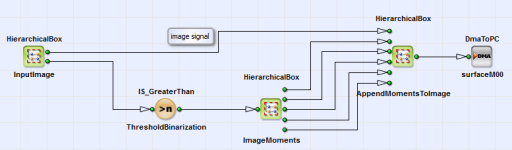Default Platform: mE5-MA-VCL

Short Description

Calculates the image moments orientation and eccentricity for the incoming images.

Moments in image processing are average values from the single pixels` intensities of an image. With this moments physical properties like orientation, eccentricity, the area or the centroid of an object in the image can be identified.

#### Orientation

The orientation of an object is defined as [Bur06]:

Equation 24.

with the central moments of second order:

Equation 25.

with definition

Equation 26.

The centroid is

Equation 27.

and the raw moments

Equation 28.

corresponds to the area of an object. Here g(x,y) is the greyscale function for digital greyscale images.

#### Eccentricity

The eccentricity e of an object can be calculated with [Bur06]

Equation 29.

The results of the eccentricity are in a range between 0 (round object) and 1 (elongated object).

In VisualApplets a design is implemented with the original image and geometric properties like the area, the center of gravity, the orientation and the eccentricity of an object as output. You can find the example under \examples\Processing\Geometry\ImageMoments\ImageMoments.va. A parallelism of 4 was chosen for calculation. In Figure 307, 'Basic design structure' the structure of the design with comments is shown.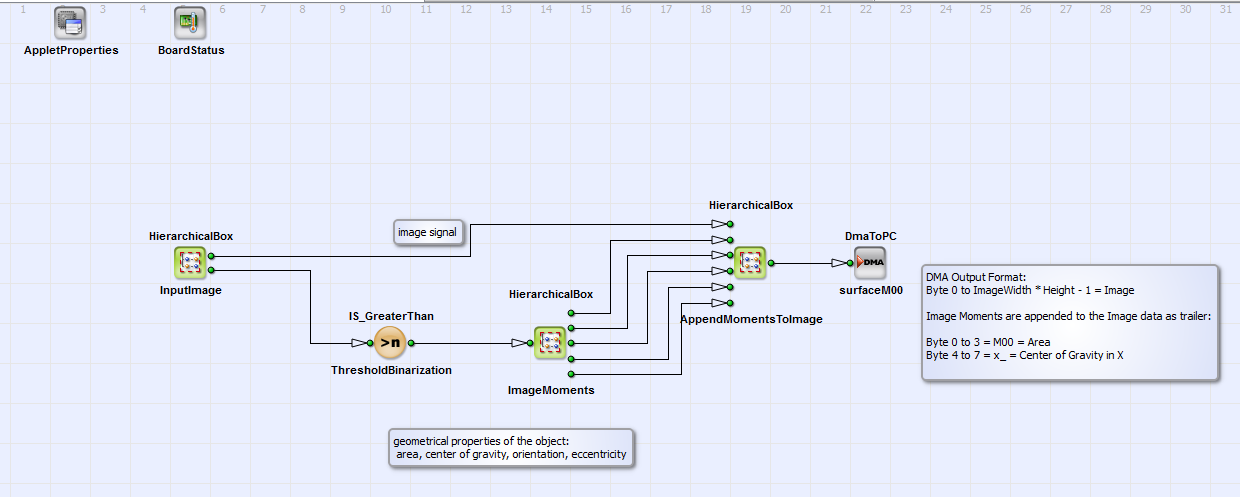Figure 307. Basic design structure

Geometric properties area, center of gravity, orientation and eccentricity of a greyscale image are calculated via moments (HierarchicalBox: ImageMoments). These properties are appended to the original camera image (AppendMomentsToImage). In Figure 308, 'Content of ImageMoments' the content of the HierarchicalBox ImageMoments is shown. The raw moments (which corresponds to the area of an object), and , as well as the center of gravity and the central moments and are implemented according to the formulas given above. Finally the geometric properties orientation of the main axis and eccentricity e of an image object are calculated from these results.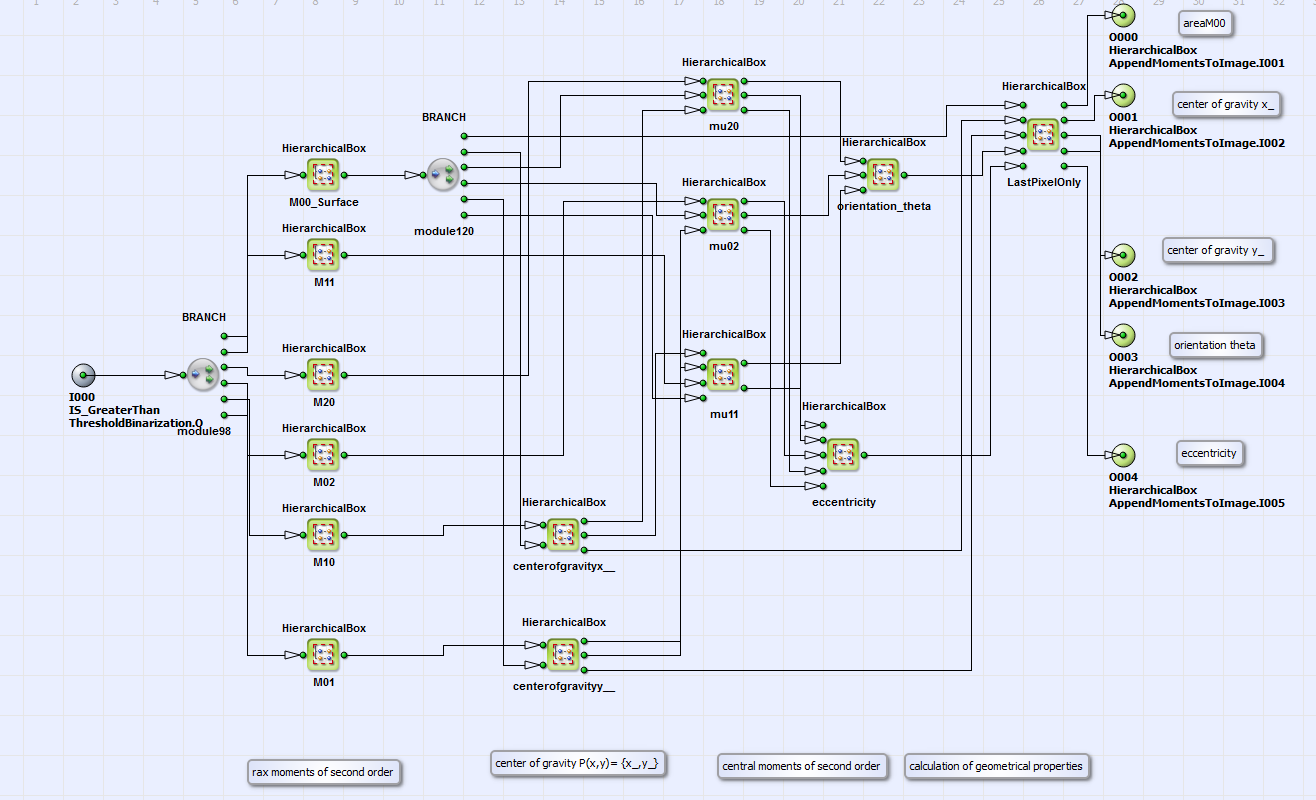Figure 308. Content of ImageMoments

In Figure 309, 'Content of the HierarchicalBox orientation_theta' and Figure 310, 'Content of the HierarchicalBox eccentricity' the contents of the HierarchicalBoxes orientation_theta and eccentricity together with inserted comments on the single operation steps are shown. The orientation and eccentricity e are calculated by using the values of according to Equation 24 and Equation 29. A resolution of R=7 is chosen for the input signal to the ARCTAN operator.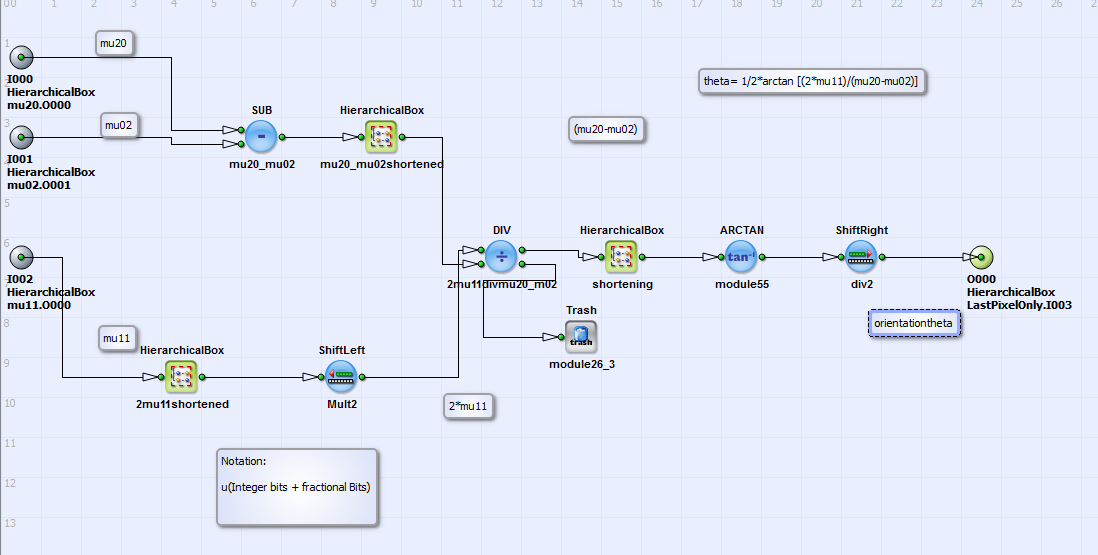Figure 309. Content of the HierarchicalBox orientation_theta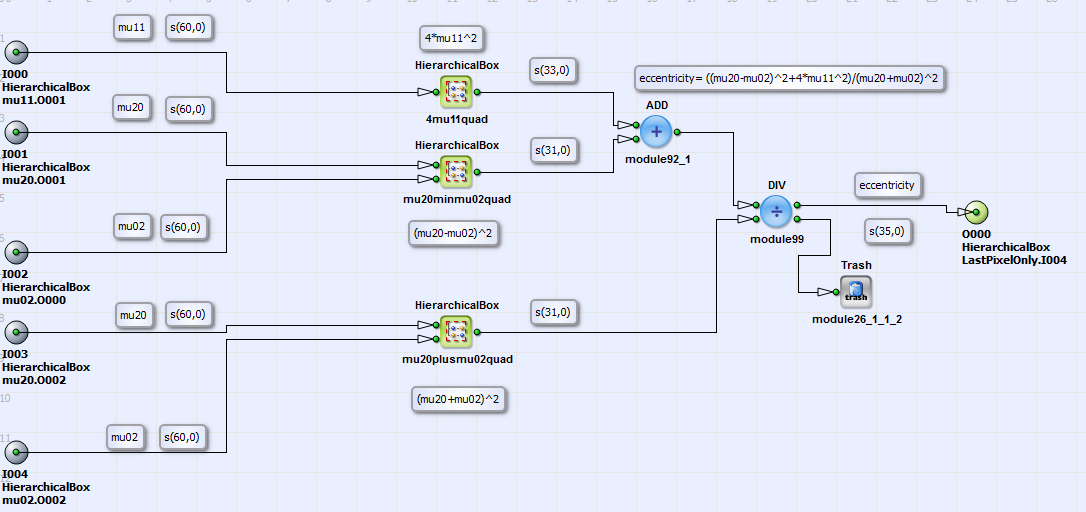Figure 310. Content of the HierarchicalBox eccentricity

Comment: During implementation of the orientation of Θ it showed, that limitation to 12 bits maximum input signal of the ARCTAN function can be a problem for calculation of accuracy and resolution: for example the image of an ellipse with orientation Θ= 44.07° (result of moment implementation with MATLAB) was analyzed. The 25 bit input signal for the ARCTAN function has to be limited to 12 bits. With resolution of R= 8 bits the implementation in VisualApplets has result 41.43°; with R=7 bits the result is 43.21° (with resolution for small angles of 0.2°) and with R= 6 it is 44.07° (with resolution for small angles of 0.45°). As an result we found that with high resolution (R= 8 bit) the maximum angle of calculation is smaller than the true angle of orientation, due to bit depth limitation. With smaller resolution R the result of calculation improves for big angles but has as an consequence smaller accuracy in the range of small angles. The problem can be solved by using a Lookup table instead of ARC TAN operator.